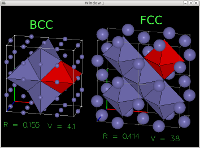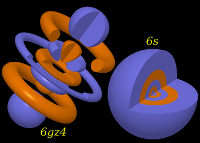GAMGI 0.16Orbitals 6hz5, 6hy5, 6s are shown here, the last one represented only by its lower half, to evidence its 5 node internal structure. Each orbital is represented as a cloud of points above a probability density of 1E-6, 300,000 points for 6hz5, 6hy5 and 3,000,000 for 6s (to properly show the inner node). Users can choose which octants of the orbital to show, allowing a detailed analysis of its internal structure. Gnome on Debian 6.0. Size: 28,703 bytes.The five 3d orbitals are shown here with the same orientation, inside a xyz frame, in orthographic projection. Each orbital is represented as a cloud of 150,000 points above a probability density of 1E-6. 3dz2 and 3dx2-y2 are ligned with the xyz axes, while 3dxy, 3dxz, 3dyz are aligned along the xyz bisectors. Gnome on Debian 6.0. Size: 25,325 bytes.Octahedral interstices are smaller in BCC (r/R = 0.155) than in FCC (r/R = 0.414) structures. However the corresponding octahedra are larger in BCC (V = 4.1 Angstron**3) than in CFC (V = 3.8 Angstron**3) structures! Gnome on Debian 6.0. Size: 91,906 bytes.6px Hydrogen orbital, represented as the outer solid isosurfaces with 1E-5 probability density, sampled with an accuracy of 150 cells per direction per octant. Gnome on Debian 6.0. Size: 116,699 bytes.6gz4 and 6s Hydrogen orbitals, represented as the outer solid isosurfaces with 1E-5 and 1E-6 probability densities, respectively. The sampling accuracy is 150 and 100 sampling cells per direction per octant, respectively. To show the inside, one and two octants have been removed in the 6gz4 and 6s orbitals, respectively. Removing arbitrary octants from the representation allow users to view the orbital inside, particularly useful in s orbitals. Gnome on Debian 6.0. Size: 126,971 bytes.

GAMGI old

Screenshots for older releases can be found here.